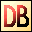DelphiBasics
 PrivateDirective Starts the section of private data and methods in a class
type Class declaration
Private
Data | Method declaration;
{...}
end;
Description
The Private directive starts the section of data (fields) and routines (methods) of a class that are private (internal) to that class.

It is a vital part of the Object Oriented concept that a class be treated like a black box - how it operates internally is not relevant to the external use. This is where the Private section comes in - it hides data and routines used solely by other routines in the class.

If private data needs to be accessed externally, a public (or published) property or routine is provided to give this access.

Private data and routines are not even accessible by a descendent class - you must use Protected to provide this access. Protected data and methods are externally invisible, but are accessible to all classes in a hierarchy.
Related commands
 Function Defines a subroutine that returns a value Procedure Defines a subroutine that does not return a value Property Defines controlled access to class fields Protected Starts a section of class private data accesible to sub-classes Public Starts an externally accessible section of a class Published Starts a published externally accessible section of a class Type Defines a new category of variable or processDownload this web site as a Windows program.

 Example code : A class with private data and a private routine // Full Unit code. // ----------------------------------------------------------- // You must store this code in a unit called Unit1 with a form // called Form1 that has an OnCreate event called FormCreate. unit Unit1; interface uses   Windows, Messages, SysUtils, Variants, Classes, Graphics, Controls, Forms,   Dialogs; type   // Class with Indexed properties   TRectangle = class   Private     fCoords: array[0..3] of Longint;     function  GetCoord(Index: Integer): Longint;     procedure SetCoord(Index: Integer; Value: Longint);   public     property Left   : Longint index 0 read GetCoord write SetCoord;     property Top    : Longint index 1 read GetCoord write SetCoord;     property Right  : Longint index 2 read GetCoord write SetCoord;     property Bottom : Longint index 3 read GetCoord write SetCoord;     property Coords[Index: Integer] : Longint read GetCoord write SetCoord;   end;   // The form class itself   TForm1 = class(TForm)     procedure FormCreate(Sender: TObject);   end; var   Form1: TForm1; implementation {\$R *.dfm} // TRectangle property 'Getter' routine function TRectangle.GetCoord(Index: Integer): Longint; begin   // Only allow valid index values   if (Index >= 0) and (Index <= 3)   then Result := fCoords[Index]   else Result := -1; end; // TRectangle property 'Setter' routine procedure TRectangle.SetCoord(Index, Value: Integer); begin   // Only allow valid index values   if (Index >= 0) and (Index <= 3)   then fCoords[Index] := Value; end; // Main line code procedure TForm1.FormCreate(Sender: TObject); var   myRect : TRectangle;   i : Integer; begin   // Create my little rectangle   myRect := TRectangle.Create;   // And set the corner coordinates   myRect.Left := 22;      // Left using direct method   myRect.Top  := 33;   myRect.SetCoord(2,44);  // Right using indexed method   myRect.SetCoord(3,55);   // And ask for these values   for i:= 0 to 3 do     ShowMessage('myRect coord '+IntToStr(i)+' = '+intToStr(myRect.GetCoord(i))); end; end. myRect coord 0 = 22   myRect coord 1 = 33   myRect coord 2 = 44   myRect coord 3 = 55